#Function Repository Resource:

# Excess01Ruler

Find a near-minimal set of integer marks so that all integers up to a given length are represented as differences

Contributed by: Ed Pegg Jr
 ResourceFunction["Excess01Ruler"][length] finds a set of integers representing all differences up to length using a compact form for the result. ResourceFunction["Excess01Ruler"][length,"Full"] finds a set of integers representing all differences up to length.

## Details and Options

The "Full" calculation requires significant memory for large length values.
An integer length ruler with marks so that all integer differences up to the length are represented is called a complete ruler.
The excess of a complete ruler with length L and M marks is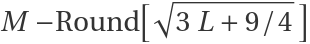.
For any positive integer length, a complete ruler with excess 0 or 1 can be made, hence these are called excess-01 rulers.
There are no known complete rulers with an excess of -1.

## Examples

### Basic Examples

Find the marks for a sparse ruler of length 9:

 In:=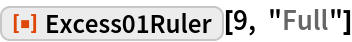Out=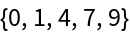Check that all differences are covered:

 In:=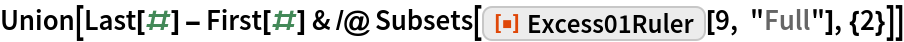Out=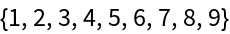Find the marks for a sparse ruler of length 57:

 In:=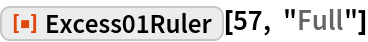Out=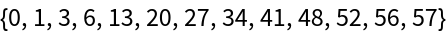Check that the ruler is complete (i.e. all differences are covered):

 In:=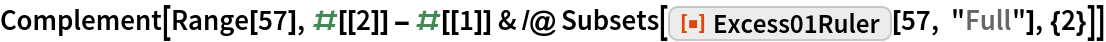Out=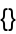Show the intervals between marks in the full form of a length-396 ruler:

 In:=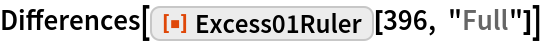Out=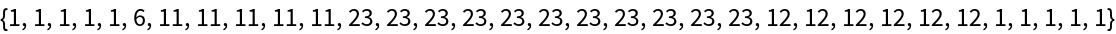Split the differences:

 In:=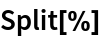Out=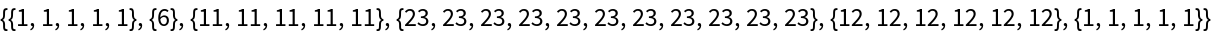Make a series of lists from the compact form of a length-396 ruler:

 In:=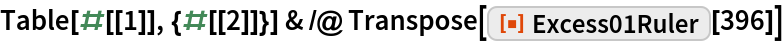Out=Show the compact form:

 In:=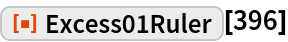Out=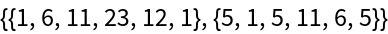Verify that the compact form from the previous result has length 396:

 In:=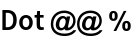Out=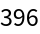Show the full form for a length-396 ruler:

 In:=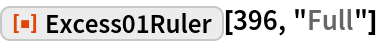Out=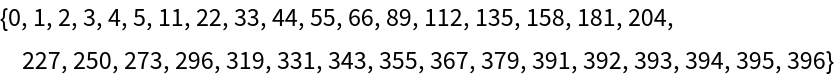### Scope

Show the marks for the first 51 rulers:

 In:=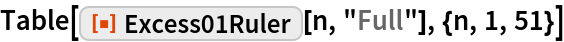Out=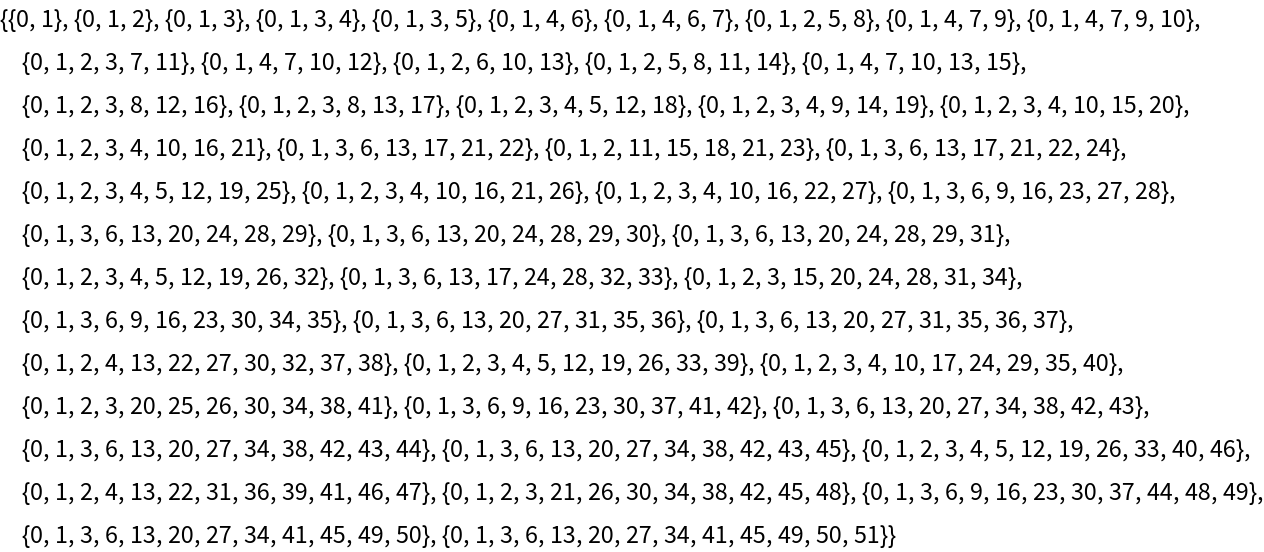Show the excess values for the first 51 rulers:

 In:=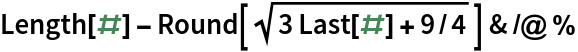Out=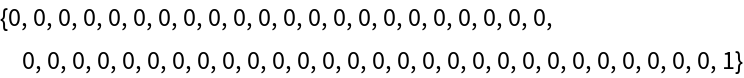Count the number of marks for a length-58 ruler in full form or compact form:

 In:=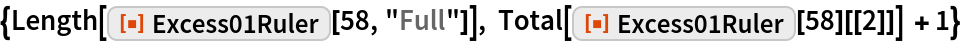Out=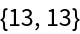The excess of a ruler with length L and M marks is:

 In:=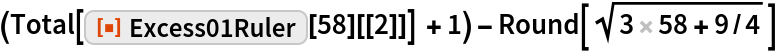Out=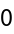An excess-01 ruler has an excess of 0 or 1:

 In:=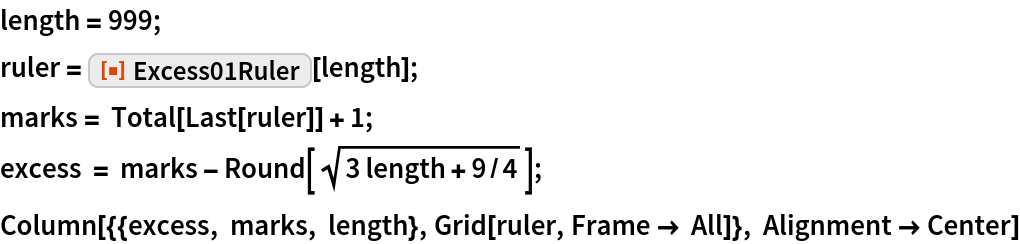Out=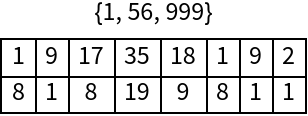A list of some rulers with a single mark less than those generated by this function: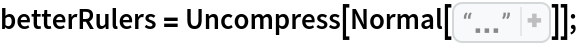Show the length-999 ruler with one less mark:

 In:=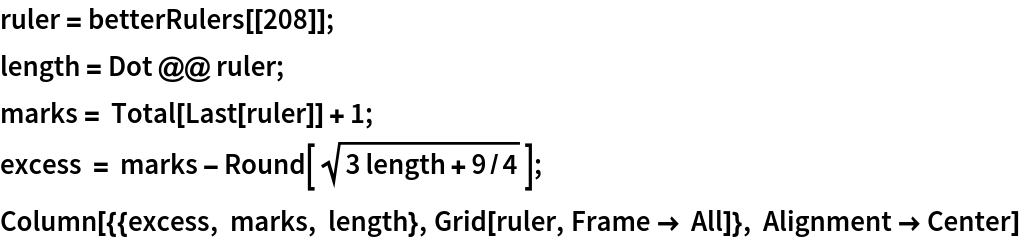Out=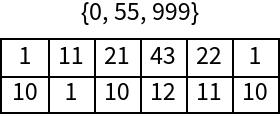Show how the Leech upper bound for the number of marks in a complete ruler compares to the number of marks in function-generated rulers:

 In:=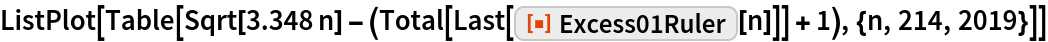Out=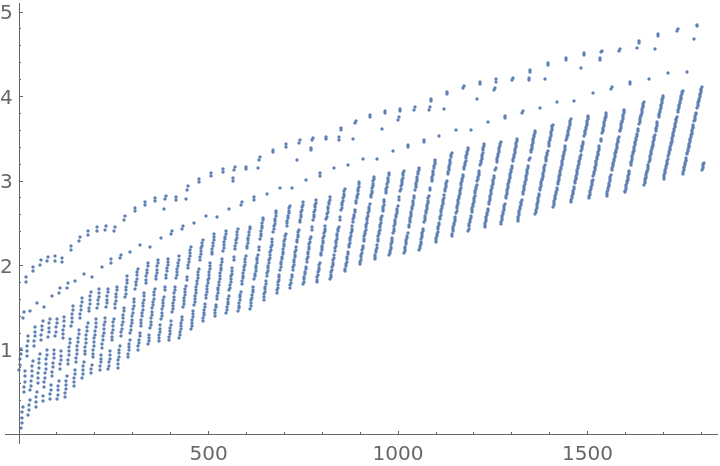Generate rulers of length n! and plot the number of marks:

 In:=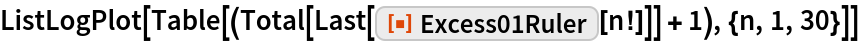Out=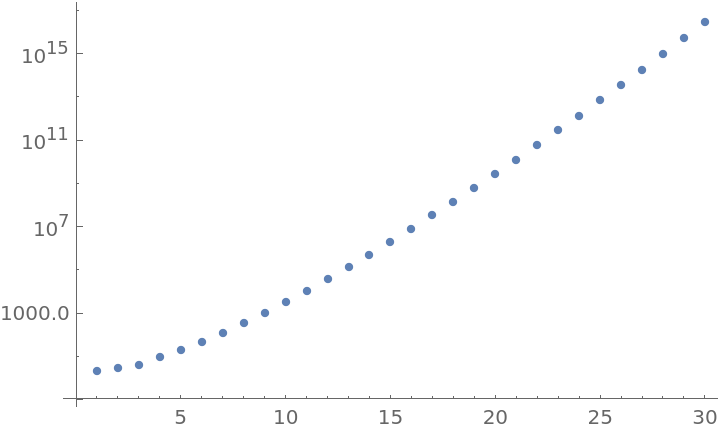### Possible Issues

Show the default shortened form for a length-googol ruler:

 In:=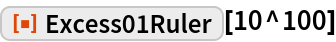Out=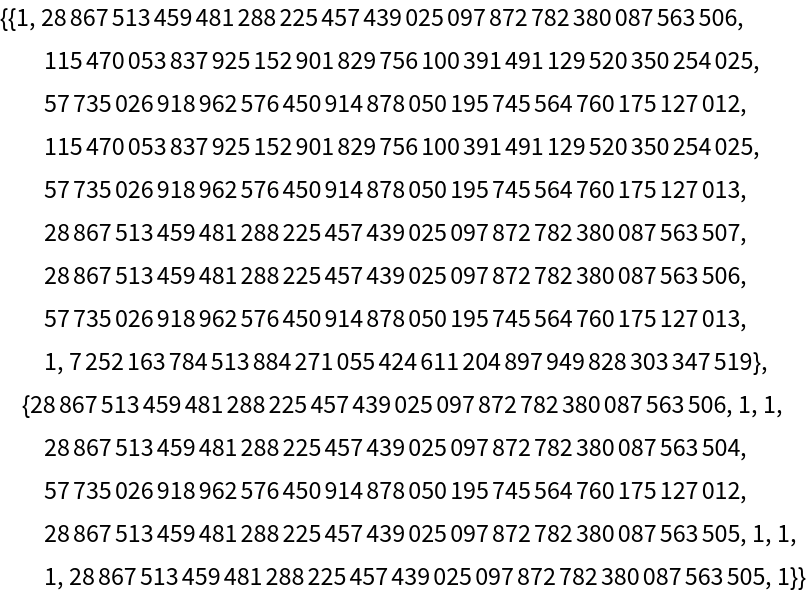Show the number of marks in a length-googol ruler:

 In:=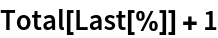Out=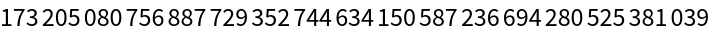Showing the full form of a googol-length ruler is not recommended.

### Neat Examples

Generate reasonable rulers for various integer powers and calculate their excess:

 In:=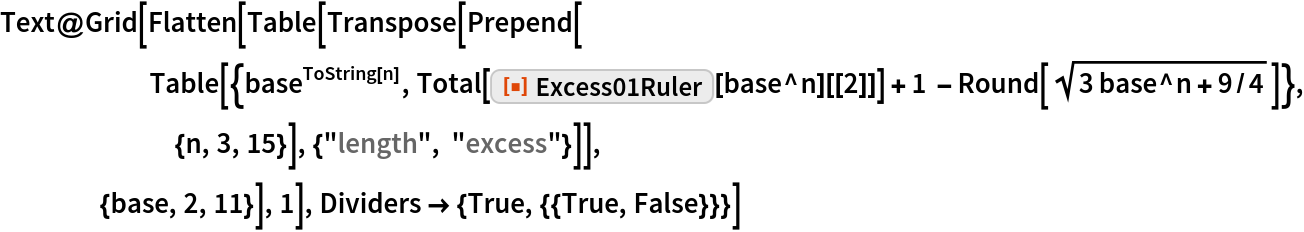Out=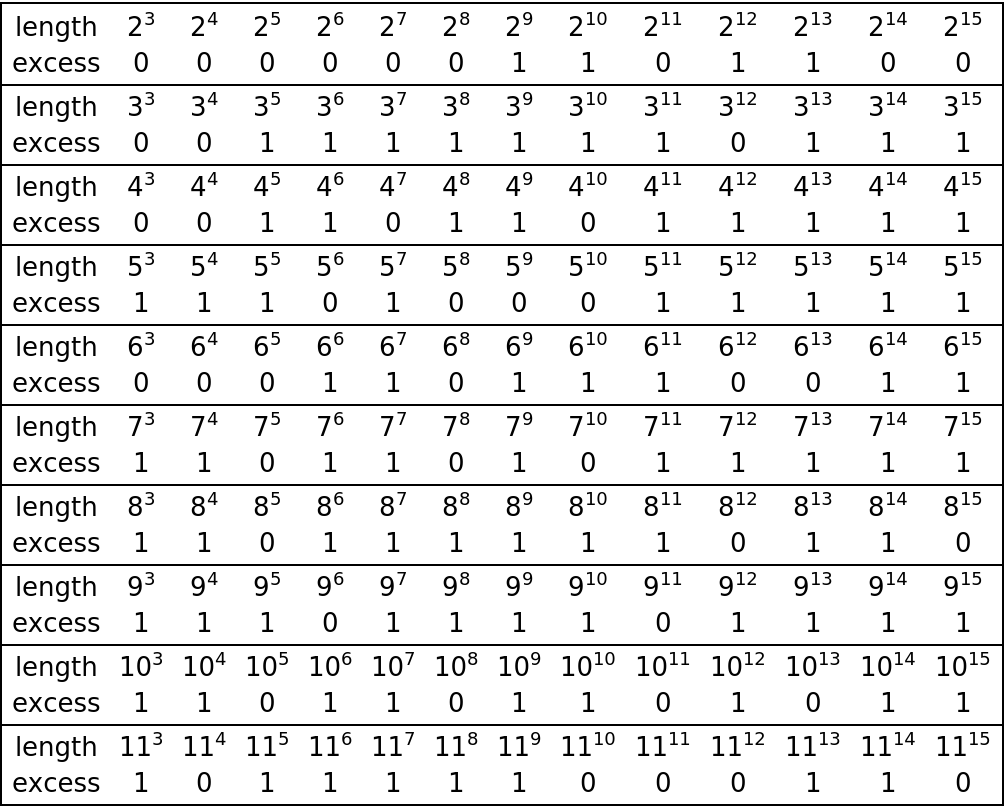All rulers to length 213 are minimal. Show a pixel representation of these sparse rulers:

 In:=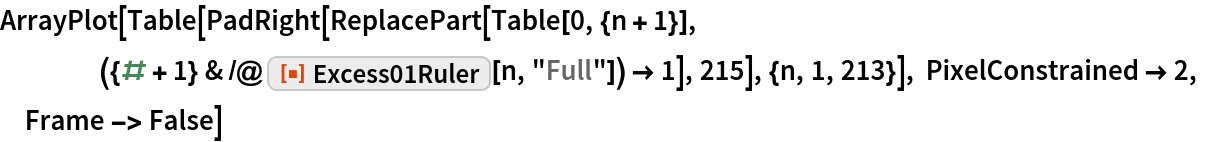Out=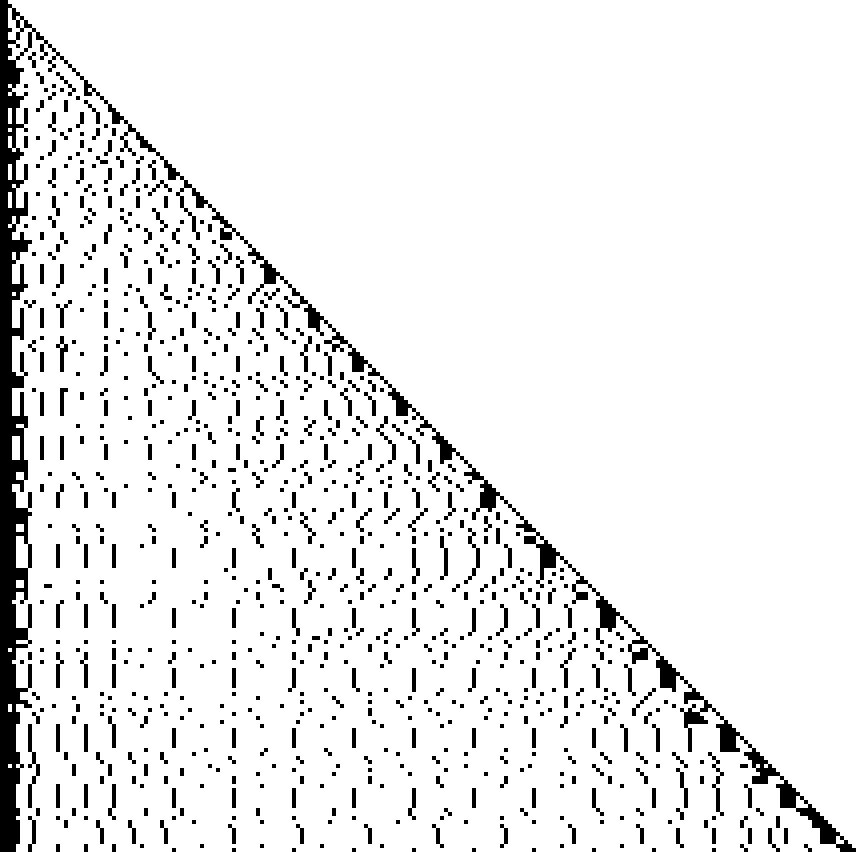The maximal length for a given number of marks is usually a Wichmann value:

 In:=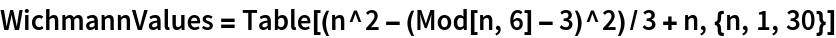Out=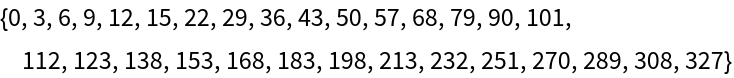Arrange lengths in columns ending in Wichmann values and bold the lengths with excess 1:

 In:=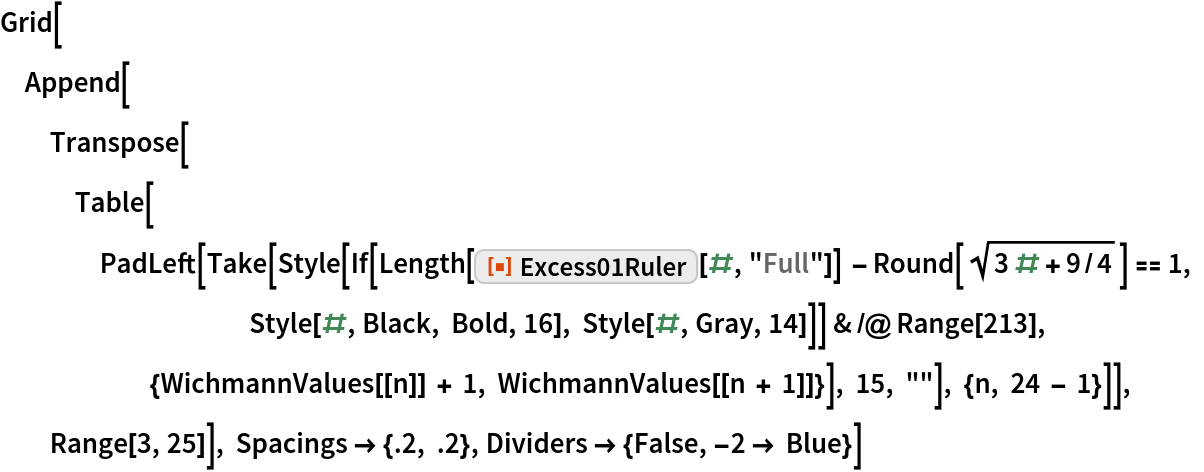Out=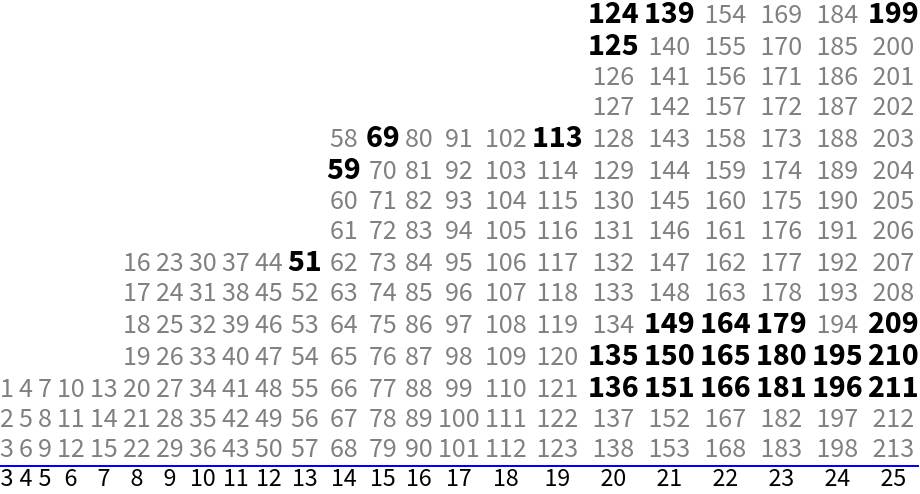## Author Notes

No sparse ruler is known with an excess less than 0.

This function has not been extensively tested for values past 20 million.